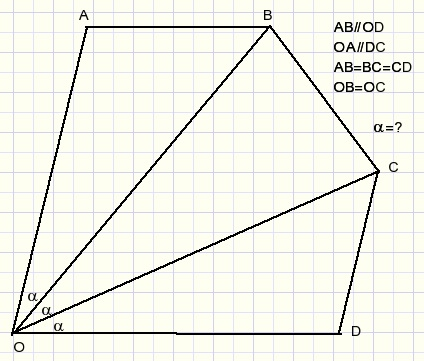Geometry problems (1..10)problems 11..20

1.
Given are:
- a circle with radius r1 and inside
- two circles with radius r2 and r3 which contact circle 1 at points A and B
- the centers of circles 1,2 and 3.
- the intersection point P of circles 2 and 3

Prove that:
If P is on line AB then r1 = r2 + r3 must be true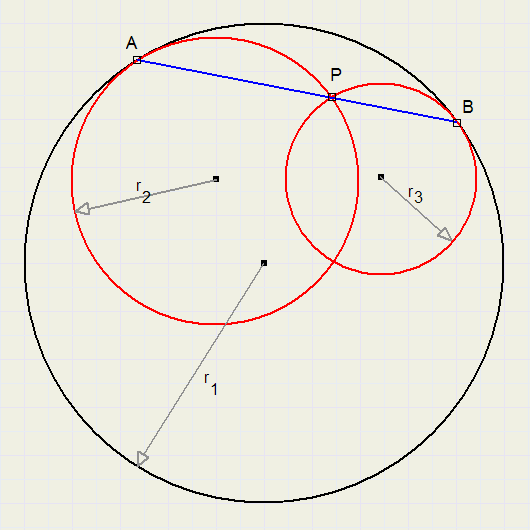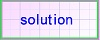2.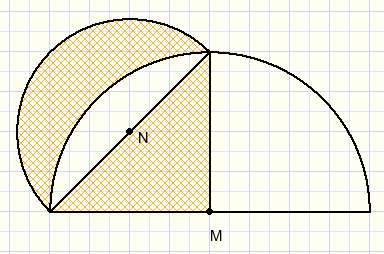In the figure above the circles have centers M, N.
Proof, that the marked areas are equal.3.Given are:
Equilateral triangle ABC.
M is the center of BC and also center of a circle arc through B and C.
Arcs CF, FG and GB are equal in length.
Prove that :
CD = DE = EB.4.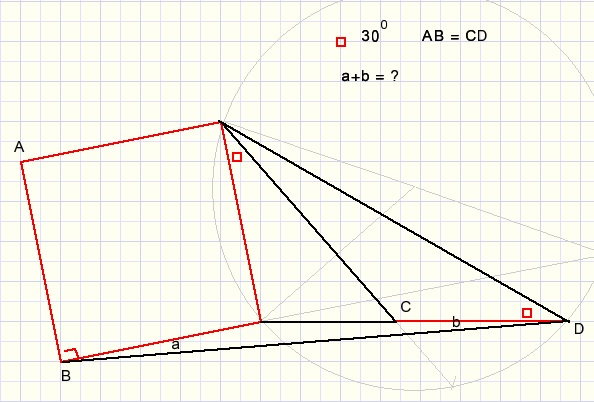Given is a square and a line CD = AB.
Some angles (indicated by a small red square) are 300
Asked is the sum of angles a+b.5.
Given are points A and B and a circle c.
Construct a circle through A and B that intersects c in diametrically opposite points.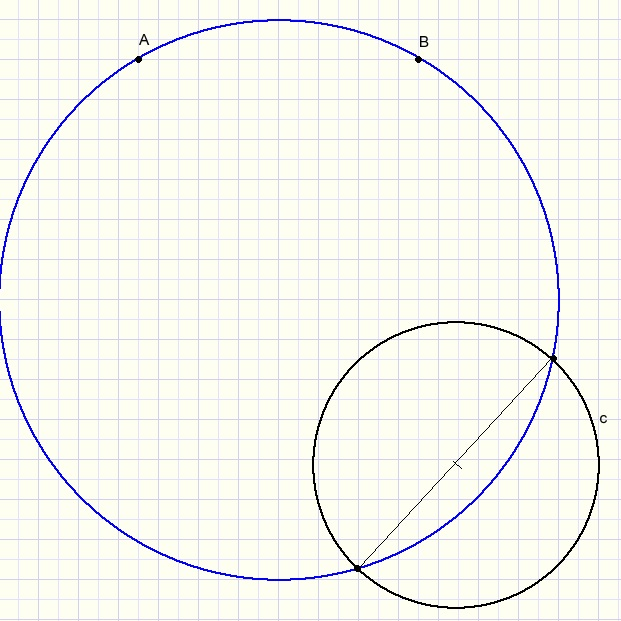6.
Given is square ABCD.
Triangle CDP is equilateral.
Calculate the size of LBAP.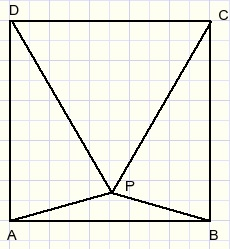7.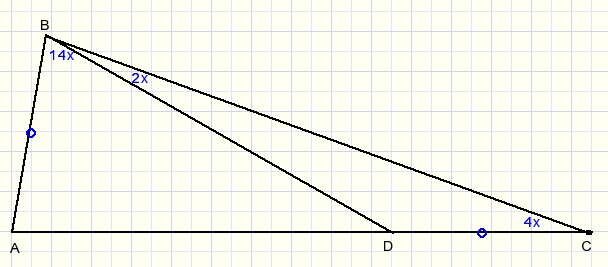Given are:
1. ΔABC with point D on BC such that AB=DC.
2. The relative size of some angles, see figure (in blue).

Asked: find the value of x.8.
Problem: calculate angle x in figure below:9.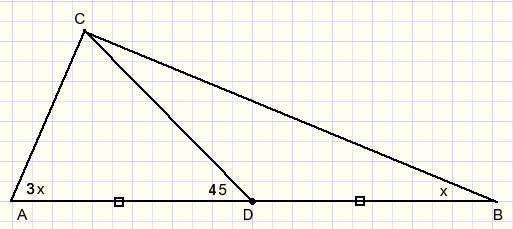Question: prove that x=22.510.